HomeBlog

# Feedback in Switching Power Supply Circuit Design

In addition to magnetic element design, feedback network design is also the least known and very troublesome work of switching power supply. It involves analog electronic technology, control theory, measurement and computing technology and other related issues.

Catalog

 Introduction Switching Power Supply Design 1. Frequency Response 1.1 Basic Concept1.2 Frequency Response of Basic Circuits 1.2.1 High Frequency Response1.2.2 Phase-frequency Characteristic1.2.3 Low Frequency Characteristic 1.3 Characteristics of LC Filter Circuit 2. Time-Domain Response of Basic Circuits 2.1 Step-function Signal2.2 Step Response of Single Time Constant2.3 Step Response of LC Circuit 3. Plural Formula explanation 4. Complex Function Formula explanation 5. Exchange C and L Formula explanation

The purpose of switching power supply loop design is to achieve the required output (voltage or current) accuracy within the range of input voltage and load variation, and meanwhile, makes equipment to work stably under any circumstances. What’s more, achieve fast response and small overshoot when load or input voltage changes. At the same time, it can reduce the low frequency pulsation component and the switch ripple and so on.

To better understand the feedback design method, the basic knowledge of frequency characteristics, negative feedback and operational amplifier in analog circuits is reviewed importantly. Here the basic design method of feedback compensation is discussed with the example of forward converter. It also introduces how to test the open loop response by using analyzer HP3562A, and then design and correct the network according to the test characteristics and verify the design results. Finally, introduce the simulation test.

1. Frequency Response

In electronic circuits, reactance (inductor and capacitor) elements are inevitable. For different frequencies, their impedance varies with frequency. Their electrical signals not only change in amplitude, but also in phase. The relation between output and input of sinusoidal signals with different frequencies is called frequency response.

1.1 Basic Concept

The output-to-input ratio of the circuit is called a transfer function or gain. The relation between the transfer function and the frequency, that is, the frequency response can be represented by the following expression: G=(f)∠φ(f), while G(f) is the relation between the modulus (amplitude) of the transfer function and the frequency, which is called the amplitude-frequency response; ∠φ(f) is the relation between the phase difference of the output signal and the input signal and frequency, which is called the phase frequency response.

The typical logarithmic amplitude-frequency response is shown in Fig. 1, and Fig. 1 (a) is the amplitude-frequency characteristic. It is drawn on the logarithmic coordinate with logarithmic frequency f as the transverse coordinate, and the longitudinal axis gain is represented by 20logG(f). Fig. 1 (b) is the phase frequency characteristic, and the vertical axis represents the phase angle φ on the single logarithmic coordinate with logarithmic frequency f as the transverse coordinate. This diagram is called Potier graphs.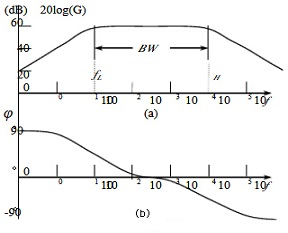Fig. 1 Potier Graphs

In terms of amplitude-frequency characteristics, there is a frequency range in which the gain is basically constant, and when the frequency is higher or below than a certain frequency, the gain will decrease. When the high frequency increases, if the gain is lower than the constant part of the 3dB, the frequency is called the upper limit frequency or the upper limit cut off frequency, representing by fH, while the frequency is larger than the cut-off frequency is called the high frequency region. At low frequency, when the gain is lower than the constant part of 3dB, the frequency is called the lower frequency or the lower rate limit, representing by fL, where the frequency is lower than the lower cut-off frequency is called the low frequency region. Between the high-frequency cut-off frequency and the low-frequency cut-off frequency is called the intermediate frequency region. In this area, The gain is basically unchanged. The definition of it: BW=fH-fL

1.2 Frequency Response of Basic Circuits

1.2.1 High Frequency Response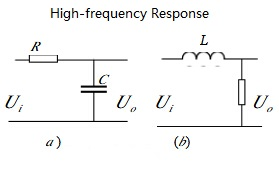Fig. 2 High- frequency Response

In the high-frequency region, the circuit that affects the high-frequency response of the system (circuit) is shown in Fig. 2. Taking Fig. 2(a) as an example, the ratio of output voltage to input voltage decreases with the increase of frequency, and meanwhile the phase lags.

Using complex variables to obtain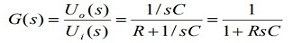As for the actual frequency, s=jw=j2πf , making(F-0)

The high-frequency voltage gain of the circuit can be obtained: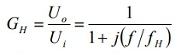The relationship between the frequency and phase angle, and the mode (amplitude) of the gain in the high frequency region are obtained: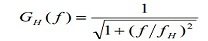The logarithmic amplitude-frequency is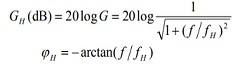(F-1)

1.2.1 Amplitude-frequency response

1) when f<<fH,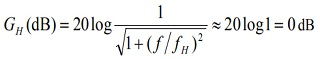The gain value is 1, a horizontal line at the horizontal coordinates;

2) when f>>fH,It can be seen that for the logarithmic frequency coordinate, the upper formula can be represented by an oblique line, the slope is -20dB/ tenth frequency (- 20dB/dec), and intersects with the 0dB line at f=fH, so fH is called turning frequency. When f=fH, that is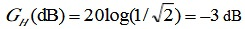, the high frequency response takes the 0dB line and-20dB/dec as the asymptote, and the maximum difference at the turning frequency is-3dB. The amplitude-frequency characteristic is shown in Fig. 3(a)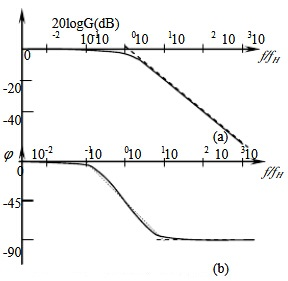Fig. 3 High- frequency Potier Diagram

When the frequency is equal to the turning frequency, the capacitor reactance is exactly equal to the resistance. When the frequency increases continuously, the impedance of capacitor C decreases by-20dB/dec, that is, the frequency increases by 10 times and the capacitive reactance decreases by 10 times, so the output attenuates with-20dB.

1.2.2 Phase-frequency Characteristic

The relationship between phase and frequency can be made in the following ways according to formula (F-2).

When f<<fH, φ closes to 0 ° , getting a straight line.

When f>>fH, φ closes to 90 ° , getting a straight line.

When f=fH, φ=45 °.

When f=0.1fH, and f=10fH, φH is -5.7 °and -84.3 °respectively, so the slope is represented approximately by 45/dec oblique line. The phase frequency characteristics are shown in the following figure.Fig. 3 High- frequency Potier Diagram

From the amplitude-frequency and phase-frequency, it can be seen that when the frequency increases, the gain of the circuit becomes smaller and the phase lag becomes larger. When the phase reaches 90 °, the gain is 0. Both amplitude-frequency and phase-frequency characteristics are determined by upper frequency fH. It can be seen from formula (F-0) that the upper cut-off frequency is determined by the time constant (RC) of the circuit. If the time constant L /R of Fig. 2(b) is equal to the time constant RC of Fig. 2(a), the porter diagram of Fig. 2(b) circuit is exactly the same as that of Fig. 2(a).

As can be seen from Fig. 3, the high frequency signal attenuates greatly, while the low frequency signal is preserved. Therefore, this circuit is also called a low-pass filter. For Fig. 2(a) circuits, if the time constant is much larger for the time studied, that is, the resistance and capacitance values are largeUo=Uc，From  it can get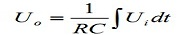This is an integrator. It can be seen that the same circuit has different functions for different research purposes.

1.2.3 Low Frequency Characteristic

We study the characteristics of the two circuits in the low frequency region shown in Fig. 4.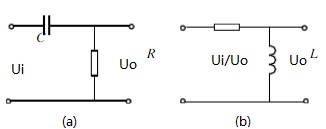Fig. 4 Low-frequency Region

Using the complex variables, from Fig. 5 (a),Fig. 5 Low- frequency potier Diagram

we can get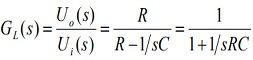According to actual frequency and s=jw, making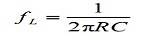Getting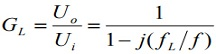Thus the gain (mode) and phase angle of the low frequency region of the circuit are respectively: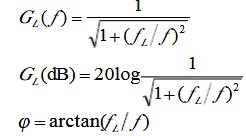Use the linear approximation method which is similar to the high frequency response, the potier diagram of the low frequency response can be drawn, as shown in Fig. 5. The fH in the diagram is the lower limit frequency, that is, the low turning frequency. Below the turning frequency, the gain of the circuit decreases with the decrease of the frequency, and the characteristic slope is 20dB/dec. When the phase reduces with the frequency, using the forward input phase. Maximum advance 90 °, gain 0 (- ∞, dB).

The lower limit transition frequency is also related to the circuit time constant RC (L/R). If the time constants of Fig.4 (a) and Fig.4 (b) are the same, their potier graphs are identical.

It can also be seen from Fig.5 that the circuit attenuates the low frequency signal, while the high frequency signal passes smoothly due to the reduction of capacitance. So this circuit is also called a high-pass filter. For Fig. 4(a), when the time constant of the Fig. 4(a) circuit is much smaller than the time interval we studied, the output obtains the variable input signal, then the circuit is a differential circuit.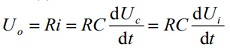1. 3 Characteristics of LC Filter Circuit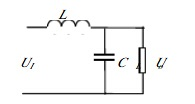Fig. 6 Frequency Characteristic of LC Filter Circuit

In the switching power supply, the forward output filter (Fig. 6) is a LC network with a load resistor in parallel with the output capacitor, and the load resistor can be changed from a certain value (full load) to infinity (no load). For Fig. 6, we can also use complex variables to get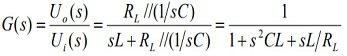According to actual frequency and s=jw, making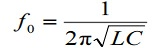Getting(F-2)

The characteristic impedance of the circuit is, at small range of f close to f0,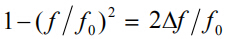, making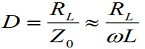, so The gain amplitude-frequency and phase-frequency characteristics are as follows respectively: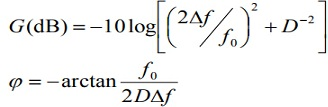(F-3)

The Potier diagram of the LC filtering circuit can be made by the expressions (F-3), as shown in Fig.. When f <f0, the formula (F-3) tends to 1, that is 0db, φ≈ 0°; When f >f 0, the second term in the denominator (F-2) is much larger than the other two, the inductive reactance is increased by 20dB/dec, the capacitive reactance by 20dB/dec is decreased, the load impedance is far greater than the capacitive reactance, and the amplitude-frequency is decreased by 40dB/dec, φ tends to -180 °. When f is close to f0, different D values and amplitudes do not increase. The greater D value is equivalent to the light load, that is circuit underdamping, the higher the amplitude.

With the increase of the load, the equivalent load resistance decreases, the D value decreases, and the peak value of lifting decreases. When D=1, at critical damping, amplitude-frequency increases slightly from low frequency to f0, at f=f0, it returns to 0dB, and when f >f0, the gain tends to -40dB/dec. When D < 1, the damping is equivalent to full load or overload. In the vicinity of f →f0, the amplitude doesn’t raise, but also attenuates with the increase of frequency, and the slope of attenuation is about 20 times of f0. The relationship between phase shift and f/fc and different D values is shown in the Fig. 8 of amplitude-frequency reaching-40dB/dec. It can be seen that the phase difference between the output and the input is 90 °at the turning frequency point f 0, regardless of the D value. For the high underdamped filter (Ro > 5Zo), the phase frequency characteristic changes rapidly with the frequency. For Ro=5Zo, when frequency at 1.5f0, the phase shift is almost 170 °. But in the circuit with gain slope of-20dB/dec, it is impossible to produce phase shift greater than 90 °, and the phase frequency characteristic changes with the frequency. The change rate of phase shift of in Fig. 8 is much lower than that of -90 °/dec in Fig. 8.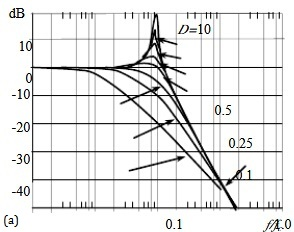Fig. 7 Frequency amplitude of LC Filter CircuitFig. 8 Phase Frequency of LC Filter Circuit

If the output capacitance in Fig. 7. has ESR , is equivalent series resistor Resr. It is generally very small and the low frequency characteristic will not be affected by 1/ωC<<Resr, in low frequency band. When the frequency increases to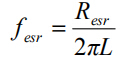At this time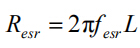,the phase is raised by 45°. As the frequency continues to rise, the output filter circuit becomes a LResr circuit. The LC filter attenuates from-40dB/dec to-20dB/dec after the frequency fesr, and the phase shift tends to lag by 90 ° instead of 180 °. This means that the capacitance of the ESR provides a zero point.

2. Time-Domain Response of Basic Circuits

The circuit analysis includes steady state analysis and transient analysis. The frequency response of the amplitude and phase of the circuit is analyzed with sine wave as the basic signal, which is the steady-state response. This method is called frequency domain analysis method.

Another method of circuit analysis is transient analysis. The step-function signal is used as input signal to study the variation of circuit output with time, which is called step response. It is judged by the rising time of the waveform and the flat-top drop size. It's called time domain analysis.

2.1 Step-function Signal

The graph represents a step voltage that can be represented as: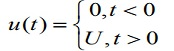It can be seen that the change rate of step signal waveform is infinite, but it is a constant during the conversion. From the point of view of frequency analysis, the extremely fast rate of change includes harmonic components from DC to very high frequency. Whether the output of the circuit can repeat the waveform of the input signal: the rising time of the output reflects the high frequency response of the circuit, while the flat top drop reflects the low frequency response of the circuit.

2. 2 Step Response of Single Time Constant

Let's study the step response of Fig. circuit. The step response is represented by the rise time tr and the flat-top landing δ.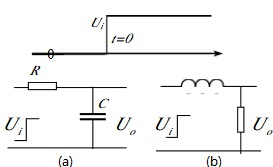Fig. 9 Step Response of Single Time Constant

Rise time tr

When the step signal is added to the input of Fig. (a) circuit, according to the general law of RC circuit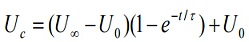U0-initial value;  U∞-terminal value; τ= RC- time constant. The capacitance initial voltage U0  is zero.In the formula τ = L/R, Ui is the voltage value of the flat top part of the step signal. The relation between Uo/Ui  and time is shown in Fig. 10. The three elements of RC circuit: initial value, final value and time constant. The input rises to the final value in a very short time, and the output voltage changes with time exponentially, which takes a period of time to reach the final value. This phenomenon is called frontier distortion. The interval between 10% of the output end value and 90% of the final value is generally defined as the rising time tr.Fig. 10 The relation between Uo/Ui and t

As can be seen from the expressions (6-18), when t=t1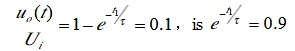according to the same principle, when t＝t2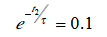Because of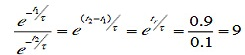So the rise time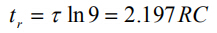High frequency response of circuit f 1/(2πRC)H，getting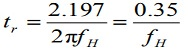Therefore, the rise time is inversely proportional to the upper bound frequency. The higher the is, the smaller the rise time tr is and the lower the front distortion is. For example, the bandwidth of a circuit is 1MHz, and the step-up time is tr=0.35 rt μs. We use Fig. (a) to study flat-top landing. When step input, the output is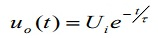The relationship between and time is shown in Fig. 11. If the time tp is small than τ, the output voltage will still decrease according to the exponential law, though the input voltage is invariable, and the decreasing speed is related to the time constant. This phenomenon is called flat-top descent.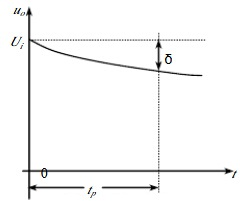Fig. 11 Flat-top Descent

Because of tp < τ, it can be approximately obtained: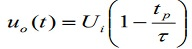Considering that fL=1/ (2πRC), then gets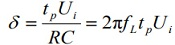It can be seen that the flat-top drop δ is proportional to the lower limit frequency fL, and the lower the fL , the smaller the flat-top fall. In switching power supply, the sudden change of load and input power supply voltage is also a step-by-step response. In the above research, the system is still in the linear state, but in the switching power supply, there are high gain amplifiers, under the action of the step signal, the system usually enters the nonlinear state, the large signal response is often lower than the small ones.

2. 3 Step Response of LC Circuit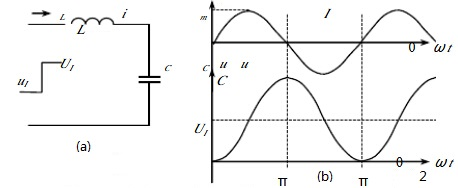Fig. 12 Step Response of LC Circuit

The LC circuit is shown in Fig. 12. If the circuit loss resistance is zero,  initial voltage of the inductance initial current and capacitance are zero, under the action of step-up signal, getting the formulas are as follows: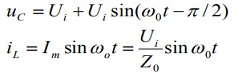Ui as step input signal; resonant angular frequency of LC circuit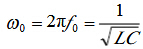Characteristic Impedance of resonant Circuit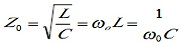The peak value of inductance current is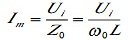Different initial values, excitation and circuit conditions, initial and final values of the waveform amplitude are different, but the phase relationship is fixed.

Note: plural concept

3. Plural

The complex number is composed of real part and imaginary part, that is,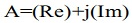, getting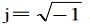Since a complex number is composed of two numbers, we can use the x axis as the real number and the y axis as the imaginary axis, as shown in Fig 13. Redraw the Fig. 13 as Fig. 14, and you can see that the complex number can be expressed in two quantities: one is the distance to the coordinates (0,0)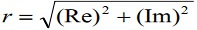, and the other is the angle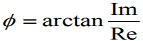from the counterclockwise to the point . The value r is called the modulus of the complex number, and the angle φ is called the amplitude angle of the complex number.Fig. 13 Complex Graphic Method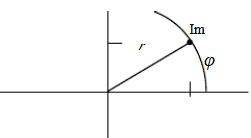Fig. 14 Expressing Complex Number by Distance and Angle

In electricity, we naturally think of using complex numbers to express values and phases. For example, if you represent a sinusoidal quantity of electricity, the sine is projected on the imaginary axis with the coordinate distance, and the cosine is projected on the real axis, so a complex number can also be represented as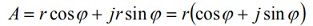(F-4)

According to Euler's formula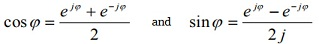The upper form can be solved as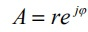(F-5), or simplified to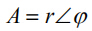(F-6)

It can be seen that a complex number can be expressed in the following ways: (F-4) is a complex cartesian coordination, (F-5) is exponential, and (F-6) is polar coordinate. The three can be converted to each other. The complex number can be added or subtracted by cartesian coordination, and the multiplication and division operations by the exponential or the polar coordinates.

According to the above mentioned formulas, if φ==90°, so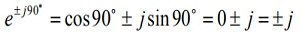Any phasor multiplied by j, phase rotation 90°: + represents counterclockwise rotation; - represents clockwise rotation. If the virtual axis is j, times j, then rotates to the solid axis to change to -1, then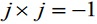, sois the imaginary unit.

4. Complex Function

The instantaneous amplitude and phase can be expressed by a complex number. If a sinusoidal quantity is expressed, the complex number in the circuit is frequency dependent. There are two aspects of interest in steady-state design: what are the parameters of a function that are zero? And where is the function infinite? These two cases represent the zeros and poles of the function respectively.

For example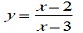It is obvious that x=2 in this function while phase is zero, that is, the complex amplitude is 2, the phase is 0, in other words, the real part is 2, and the imaginary part is 0 (Fig. 15), and the x=3 function becomes infinite. Its complex image value 3 and phase value 0 as another example, we can see that the capacitance has frequency dependent complex 1/sC (s as an complex variable, frequency-dependent), while the inductance is sL. Fig. 15 shows the switching power output filter (capacitor has ESR, inductor has coil resistance, not considered here). Form a voltage divider with an output to input ratio of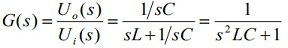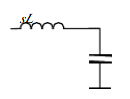Fig. 15 Complex Impedance of Inductor and Capacitor

This function will not be zero, but when, that is, there are two poles. The two poles appear at the resonant frequency point and the phase angles are 90 °and 270 °(pure imaginary number, no real part, as shown in Fig. 16 ). Of course, the physical meaning here is that the LC network resonates at this frequency and the output is amplified infinitely at this frequency. In fact, there is always resistance in the actual circuit, so the magnification is not infinite, that is, the two poles are not on the virtual axis and the real part is not zero.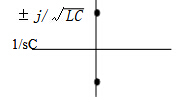Fig. 16 Poles of LC Resonant Frequency

5. Exchange C and L

For capacitive current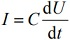If Us=Uest，the voltage is a sine wave [because of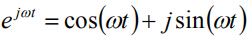]，we can get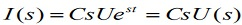Getting the resistance is: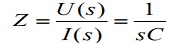In the definition of Laplace transformation, we do not have to actually solve the integral because the integral is implicit in solving the differential equation. Similarly, we can get the inductance impedance: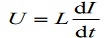Similarly, use  to replace  to get: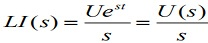So the resistance is Z=sL

### Related PostsHOW TO BUY ELECTRONIC COMPONENTS
Search
Inquiry
Order
Track

Delivery
FedEx
UPS
DHL
TNT

Payment Terms
By PayPal
By Credit Card
By Wire Transfer
By Western Union

After-sales Service
Quality Control
Guarantee
Return & Replacement